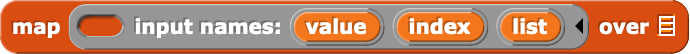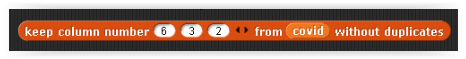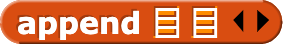# List of numbers all distincts

Hi everybody,

I have a list of lists of n numbers and I want to keep in this list only lists in which numbers are differents.
How can I do please ? Using keep items I guess but there are not enough examples in the manual and I don't really understand how to use it.

Example :

Thank you.

In your example, do you want just the lists that have 1,2,3 in some order?

Exactly.

What you're looking for is the permutations of the iota 3,iota 3,iota 3. I'll try to make that, but as I'm on my phone, it'll be hard.

Yes, this is it. I need it for a matrix project that I am actually developping. I want to calculate the determinant of a matrix without using a recursive definition, and I need to create a set af all the permutations on the dimension of the square matrix.

I'm going to see if I can find the Python version, then port that to Snap!.

i have something for you, wait a minute...

Thank you. What I just need is to create all the permutations of order n.

For that, and for other things, I'm looking for some examples of how to useon a list of lists.

try this custom blockI answered yes. They are the permutations of order n.

(edit)

Thanks but it doesn't work. Moreover, I prefer to have some explanations of how to use the blocks map, keep items with their full options.
So I shall be able to use them another time.

So if I could simply create a function with a list in entry, that reports true if all the elements are distincts, false if not, this is won.

The tricky parts are, first, the return value in the base case: There is one permutation of the empty set of values, namely an empty list. And second, you have to use APPEND to flatten the results because otherwise you'd get a list of all the permutations starting with 1, then a list of all the permutations starting with 2, etc.

That's pretty compact! I would never have thought to do that.

Thank you @bh.
But I don't know this bloc :Drag a block over the arrows of an APPEND block and it should have a red halo.

It's theblock, but (as for any variadic input) with a list dropped over the arrowheads instead of over one list slot.

Moreover, I'm trying to create a non recursive function permutations. Because recursive functions take too much time to execute.
With the bloc map, and some booleans.

Except LIST and SCRIPT VARIABLES.

That's going to be tricky, because you're expecting an output whose size is exponential in the size of the input, and the higher order functions don't explode their inputs like that. (Of course we could build more HOFs that would be specialized for this case -- but they'd have to be implemented recursively.)